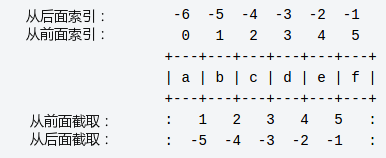# 002-Python3-基础语法-赋值、显示类型、数据类型[数值、字符串、列表、元祖、集合、字典]

基础数据类型

Python 中的变量不需要声明。每个变量在使用前都必须赋值，变量赋值以后该变量才会被创建。

在 Python 中，变量就是变量，它没有类型，我们所说的"类型"是变量所指的内存中对象的类型。

等号（=）用来给变量赋值。

等号（=）运算符左边是一个变量名,等号（=）运算符右边是存储在变量中的值。

通过赋值，确定数据类型

## 1.1、赋值

a = b = c = 1
a, b, c = 1, 2, "runoob"

## 1.2、Python3 的六个标准数据类型

• 不可变数据（3 个）：Number（数字）、String（字符串）、Tuple（元组）；
• 可变数据（3 个）：List（列表）、Dictionary（字典）、Set（集合）。

## 1.3、类型展示

内置的 type() 函数可以用来查询变量所指的对象类型。用 isinstance 来判断

>>> a, b, c, d = 20, 5.5, True, 4+3j
>>> print(type(a), type(b), type(c), type(d))
<class 'int'> <class 'float'> <class 'bool'> <class 'complex'>

>>>a = 111
>>> isinstance(a, int)
True 

区别：

• type()不会认为子类是一种父类类型。
• isinstance()会认为子类是一种父类类型。

## 1.4、删除对象引用

del 删除数值对象引用

del var_a, var_b

## 1.5、数值

python中数字有四种类型：整数、布尔型、浮点数和复数。

• int (整数), 如 1, 只有一种整数类型 int，表示为长整型，没有 python2 中的 Long。
• bool (布尔), 如 True。注意：在 Python2 中是没有布尔型的，它用数字 0 表示 False，用 1 表示 True。到 Python3 中，把 True 和 False 定义成关键字了，但它们的值还是 1 和 0，它们可以和数字相加。
• float (浮点数), 如 1.23、3E-2
• complex (复数), 如 1 + 2j、 1.1 + 2.2j

1、类型转换

• int(x) 将x转换为一个整数。

• float(x) 将x转换到一个浮点数。

• complex(x) 将x转换到一个复数，实数部分为 x，虚数部分为 0。

• complex(x, y) 将 x 和 y 转换到一个复数，实数部分为 x，虚数部分为 y。x 和 y 是数字表达式。

2、在交互模式中，最后被输出的表达式结果被赋值给变量

1 >>> tax = 12.5 / 100
2 >>> price = 100.50
3 >>> price * tax
4 12.5625
5 >>> price + _
6 113.0625
7 >>> round(_, 2)
8 113.06

### 数学函数

abs(x) 返回数字的绝对值，如abs(-10) 返回 10
ceil(x) 返回数字的上入整数，如math.ceil(4.1) 返回 5

cmp(x, y)

exp(x) 返回e的x次幂(ex),如math.exp(1) 返回2.718281828459045
fabs(x) 返回数字的绝对值，如math.fabs(-10) 返回10.0
floor(x) 返回数字的下舍整数，如math.floor(4.9)返回 4
log(x) 如math.log(math.e)返回1.0,math.log(100,10)返回2.0
log10(x) 返回以10为基数的x的对数，如math.log10(100)返回 2.0
max(x1, x2,...) 返回给定参数的最大值，参数可以为序列。
min(x1, x2,...) 返回给定参数的最小值，参数可以为序列。
modf(x) 返回x的整数部分与小数部分，两部分的数值符号与x相同，整数部分以浮点型表示。
pow(x, y) x**y 运算后的值。
round(x [,n]) 返回浮点数x的四舍五入值，如给出n值，则代表舍入到小数点后的位数。
sqrt(x) 返回数字x的平方根。

### 随机数函数

choice(seq) 从序列的元素中随机挑选一个元素，比如random.choice(range(10))，从0到9中随机挑选一个整数。
randrange ([start,] stop [,step]) 从指定范围内，按指定基数递增的集合中获取一个随机数，基数默认值为 1
random() 随机生成下一个实数，它在[0,1)范围内。
seed([x]) 改变随机数生成器的种子seed。如果你不了解其原理，你不必特别去设定seed，Python会帮你选择seed。
shuffle(lst) 将序列的所有元素随机排序
uniform(x, y) 随机生成下一个实数，它在[x,y]范围内。

### 三角函数

acos(x) 返回x的反余弦弧度值。
asin(x) 返回x的反正弦弧度值。
atan(x) 返回x的反正切弧度值。
atan2(y, x) 返回给定的 X 及 Y 坐标值的反正切值。
cos(x) 返回x的弧度的余弦值。
hypot(x, y) 返回欧几里德范数 sqrt(x*x + y*y)。
sin(x) 返回的x弧度的正弦值。
tan(x) 返回x弧度的正切值。
degrees(x) 将弧度转换为角度,如degrees(math.pi/2) ， 返回90.0

### 数学常量

pi 数学常量 pi（圆周率，一般以π来表示）
e 数学常量 e，e即自然常数（自然常数）。

## 1.6、字符串

• python中，单引号 ' 或双引号 " 括起来，同时使用反斜杠 \ 转义特殊字符。单引号和双引号使用完全相同。
• 使用三引号('''或""")可以指定一个多行字符串。
• 反斜杠可以用来转义，使用r可以让反斜杠不发生转义。。 如 r"this is a line with \n" 则\n会显示，并不是换行。
• 按字面意义级联字符串，如"this " "is " "string"会被自动转换为this is string。
• 字符串可以用 + 运算符连接在一起，用 * 运算符重复。
• Python 中的字符串有两种索引方式，从左往右以 0 开始，从右往左以 -1 开始。
• Python中的字符串不能改变。
• Python 没有单独的字符类型，一个字符就是长度为 1 的字符串。
• 字符串的截取的语法格式如下：变量[头下标:尾下标:步长]
• 索引值以 0 为开始值，-1 为从末尾的开始位置。str='Runoob'

print(str)                 # 输出字符串
print(str[0:-1])           # 输出第一个到倒数第二个的所有字符
print(str)              # 输出字符串第一个字符
print(str[2:5])            # 输出从第三个开始到第五个的字符
print(str[2:])             # 输出从第三个开始后的所有字符
print(str * 2)             # 输出字符串两次
print(str + '你好')        # 连接字符串

print('------------------------------')

print('hello\nrunoob')      # 使用反斜杠(\)+n转义特殊字符
print(r'hello\nrunoob')     # 在字符串前面添加一个 r，表示原始字符串，不会发生转义

Runoob
Runoo
R
noo
noob
RunoobRunoob
Runoob你好
------------------------------
hello
runoob
hello\nrunoob

1、格式化

print ("我叫 %s 今年 %d 岁!" % ('小明', 10)) #我叫 小明 今年 10 岁!

2、Python 的字符串常用内建函数如下：

1

capitalize()  将字符串的第一个字符转换为大写

2

center(width, fillchar)  返回一个指定的宽度 width 居中的字符串，fillchar 为填充的字符，默认为空格。

3

count(str, beg= 0,end=len(string))  返回 str 在 string 里面出现的次数，如果 beg 或者 end 指定则返回指定范围内 str 出现的次数

4

bytes.decode(encoding="utf-8", errors="strict")

Python3 中没有 decode 方法，但我们可以使用 bytes 对象的 decode() 方法来解码给定的 bytes 对象，这个 bytes 对象可以由 str.encode() 来编码返回。
5 以 encoding 指定的编码格式编码字符串，如果出错默认报一个ValueError 的异常，除非 errors 指定的是'ignore'或者'replace'
6

endswith(suffix, beg=0, end=len(string))

7

expandtabs(tabsize=8)  把字符串 string 中的 tab 符号转为空格，tab 符号默认的空格数是 8 。

8

find(str, beg=0, end=len(string))

9

index(str, beg=0, end=len(string))  跟find()方法一样，只不过如果str不在字符串中会报一个异常.

10

isalnum()  如果字符串至少有一个字符并且所有字符都是字母或数字则返 回 True,否则返回 False

11

isalpha()  如果字符串至少有一个字符并且所有字符都是字母则返回 True, 否则返回 False

12

isdigit()  如果字符串只包含数字则返回 True 否则返回 False..

13

islower()  如果字符串中包含至少一个区分大小写的字符，并且所有这些(区分大小写的)字符都是小写，则返回 True，否则返回 False

14

isnumeric()  如果字符串中只包含数字字符，则返回 True，否则返回 False

15

isspace()  如果字符串中只包含空白，则返回 True，否则返回 False.

16

istitle()  如果字符串是标题化的(见 title())则返回 True，否则返回 False

17

isupper()  如果字符串中包含至少一个区分大小写的字符，并且所有这些(区分大小写的)字符都是大写，则返回 True，否则返回 False

18

join(seq)  以指定字符串作为分隔符，将 seq 中所有的元素(的字符串表示)合并为一个新的字符串

19

len(string)  返回字符串长度

20

ljust(width[, fillchar])  返回一个原字符串左对齐,并使用 fillchar 填充至长度 width 的新字符串，fillchar 默认为空格。

21

lower()  转换字符串中所有大写字符为小写.

22

lstrip()  截掉字符串左边的空格或指定字符。

23

maketrans()

24

max(str)  返回字符串 str 中最大的字母。

25

min(str)  返回字符串 str 中最小的字母。

26

replace(old, new [, max])  把 将字符串中的 str1 替换成 str2,如果 max 指定，则替换不超过 max 次。

27

rfind(str, beg=0,end=len(string))  类似于 find()函数，不过是从右边开始查找.

28

rindex( str, beg=0, end=len(string))  类似于 index()，不过是从右边开始.

29

rjust(width,[, fillchar])  返回一个原字符串右对齐,并使用fillchar(默认空格）填充至长度 width 的新字符串

30

rstrip()  删除字符串字符串末尾的空格.

31

split(str="", num=string.count(str))  num=string.count(str)) 以 str 为分隔符截取字符串，如果 num 有指定值，则仅截取 num+1 个子字符串

32

splitlines([keepends])

33

startswith(substr, beg=0,end=len(string))

34

strip([chars])  在字符串上执行 lstrip()和 rstrip()

35

swapcase()  将字符串中大写转换为小写，小写转换为大写

36

title()  返回"标题化"的字符串,就是说所有单词都是以大写开始，其余字母均为小写(见 istitle())

37

translate(table, deletechars="")  根据 str 给出的表(包含 256 个字符)转换 string 的字符, 要过滤掉的字符放到 deletechars 参数中

38

upper()   转换字符串中的小写字母为大写

39

zfill (width)  返回长度为 width 的字符串，原字符串右对齐，前面填充0

40

isdecimal()  检查字符串是否只包含十进制字符，如果是返回 true，否则返回 false。

## 1.7、列表

list = [ 'abcd', 786 , 2.23, 'runoob', 70.2 ]
tinylist = [123, 'runoob']

1、删除

del list

2、函数

list(seq)  将元组转换为列表

3、方法

1 list.append(obj)  在列表末尾添加新的对象
2 list.count(obj)  统计某个元素在列表中出现的次数
3 list.extend(seq)

4 list.index(obj)  从列表中找出某个值第一个匹配项的索引位置
5 list.insert(index, obj)  将对象插入列表
6 list.pop([index=-1])

7 list.remove(obj)  移除列表中某个值的第一个匹配项
8 list.reverse()  反向列表中元素
9 list.sort( key=None, reverse=False)  对原列表进行排序
10 list.clear()  清空列表
11 list.copy()  复制列表

## 1.8、元组

tuple = ( 'abcd', 786 , 2.23, 'runoob', 70.2  )
tinytuple = (123, 'runoob')

tup1 = ()    # 空元组
tup2 = (20,) # 一个元素，需要在元素后添加逗号

string、list 和 tuple 都属于 sequence（序列）。

1、删除

2、函数

tuple(seq)： 将列表转换为元组。

## 1.9、集合

parame = {value01,value02,...}

set(value)

student = {'Tom', 'Jim', 'Mary', 'Tom', 'Jack', 'Rose'}

print(student)   # 输出集合，重复的元素被自动去掉

# 成员测试
if 'Rose' in student :
print('Rose 在集合中')
else :
print('Rose 不在集合中')

# set可以进行集合运算
b = set('alacazam')

print(a)
print(a - b)     # a 和 b 的差集
print(a | b)     # a 和 b 的并集
print(a & b)     # a 和 b 的交集
print(a ^ b)     # a 和 b 中不同时存在的元素

{'Mary', 'Jim', 'Rose', 'Jack', 'Tom'}
Rose 在集合中
{'b', 'a', 'c', 'r', 'd'}
{'b', 'd', 'r'}
{'l', 'r', 'a', 'c', 'z', 'm', 'b', 'd'}
{'a', 'c'}
{'l', 'r', 'z', 'm', 'b', 'd'}

clear() 移除集合中的所有元素
copy() 拷贝一个集合
difference() 返回多个集合的差集
difference_update() 移除集合中的元素，该元素在指定的集合也存在。
intersection() 返回集合的交集
intersection_update() 返回集合的交集。
isdisjoint() 判断两个集合是否包含相同的元素，如果没有返回 True，否则返回 False。
issubset() 判断指定集合是否为该方法参数集合的子集。
issuperset() 判断该方法的参数集合是否为指定集合的子集
pop() 随机移除元素
remove() 移除指定元素
symmetric_difference() 返回两个集合中不重复的元素集合。
symmetric_difference_update() 移除当前集合中在另外一个指定集合相同的元素，并将另外一个指定集合中不同的元素插入到当前集合中。
union() 返回两个集合的并集
update() 给集合添加元素

## 1.10、字典

dict = {}
dict['one'] = "1 - 菜鸟教程"
dict     = "2 - 菜鸟工具"

tinydict = {'name': 'runoob','code':1, 'site': 'www.runoob.com'}

print (dict['one'])       # 输出键为 'one' 的值
print (dict)           # 输出键为 2 的值
print (tinydict)          # 输出完整的字典
print (tinydict.keys())   # 输出所有键
print (tinydict.values()) # 输出所有值

1 - 菜鸟教程
2 - 菜鸟工具
{'name': 'runoob', 'code': 1, 'site': 'www.runoob.com'}
dict_keys(['name', 'code', 'site'])
dict_values(['runoob', 1, 'www.runoob.com'])

>>>dict([('Runoob', 1), ('Google', 2), ('Taobao', 3)])
{'Taobao': 3, 'Runoob': 1, 'Google': 2}

>>> {x: x**2 for x in (2, 4, 6)}
{2: 4, 4: 16, 6: 36}

{'Runoob': 1, 'Google': 2, 'Taobao': 3}

1、删除

del dict['Name'] # 删除键 'Name'
dict.clear()     # 清空字典
del dict         # 删除字典

2、函数

str(dict)  输出字典，以可打印的字符串表示。

type(variable)  返回输入的变量类型，如果变量是字典就返回字典类型。

3、方法

5 key in dict 如果键在字典dict里返回true，否则返回false